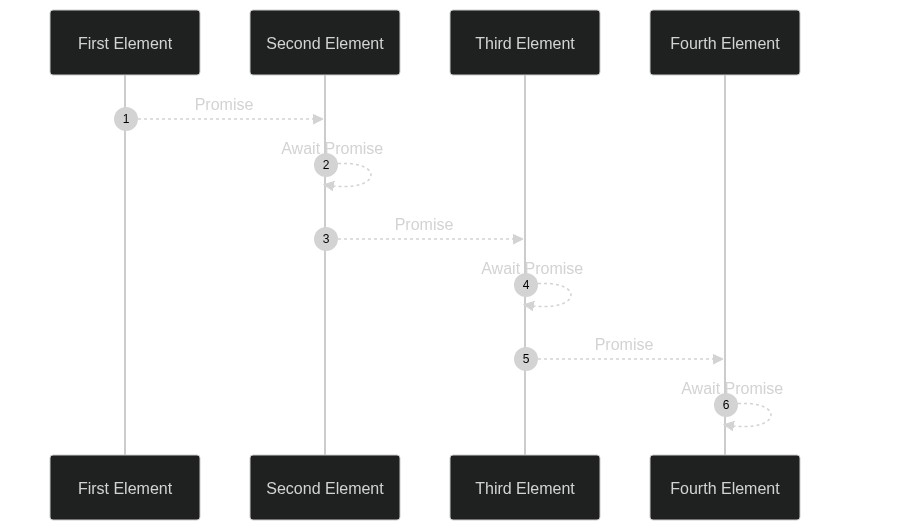Yash Singh# Working with `Array#reduce` Asynchronously

While working on a chrome extension that groups tabs together by certain criterias, I ran across a problem where I was using `Array#reduce` and had to run an asynchronous chrome extension API inside it. In this article, I will show you how to do asynchronous operations inside `Array#reduce`.

## What is `Array#reduce`?

`Array#reduce` allows you to iterate through an array and modify an accumalator object every iteration, that is provided back to the next element in the next iteration.

## Example with `Array#reduce`

The following code will run the function every iteration and pass it the current element's value and the current accumalator's value. The default accumalator is set to 0.

``````// Find the sum of an array
console.log(
[1, 2, 3].reduce(
function (accumalator, number) {
// function to run every iteration
return accumalator + number;
},
0 // accumalator value
)
);

// Expected output: 6``````

## How do you use `Array#reduce` asynchronously

This diagram might help:Basically, what happens is that every iteration, you do the asynchronous work, and return the promise. In the next iteration, the promise is resolved, and then it is processed as an accumalator. In the end, `Array#reduce` will return a promise which would resolve to the final result. Here is an example:

``````// Waits half a second then returns the sum of the two given numbers
async function sum(a, b) {
await new Promise((resolve) => setTimeout(resolve, 500));
return a + b;
}

[1, 2, 3].reduce(async (acc, num) => {
acc = await acc;
const newValue = await sum(acc, num);
return newValue;
}, 0);

// Expected: Promise<6>``````

Over here, we iterate over the array of the first three natural numbers and then define an asynchronous arrow function that first runs `await` on the `acc` and then processes it, returning the new value. The little complicated part is why we can't just remove line 8 where we await the accumalator.

It might seem like this code will work because we already run await on line 3:

``const newValue = await sum(acc, num);``

However, `Array#reduce` doesn't have any special logic to handle `async` functions, so it just evaluates it like a regular function and sets the value of the accumalator to the promise returned by the asynchronous function. That means that when our function recieves its accumalator, it recieves a promise that has to be resolved first.

For example, if we removed the await when assigning `newValue`:

``const newValue = sum(acc, num);``

To remove the await when assigning `newValue`, the next iteration will get a promise resolving to a promise.